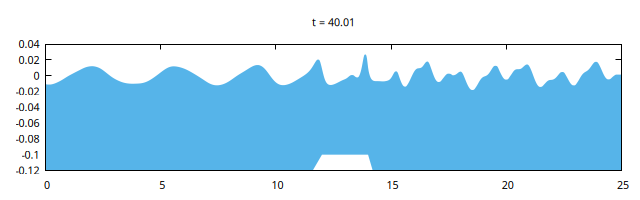# Sinusoidal wave propagation over a bar

Beji and Battjes, 1993 and Luth et al, 1994 studied experimentally the transformation of sinusoidal waves propagating over a submerged bar (or reef). This is a good test case for dispersive models as higher harmonics are nonlinearly generated and released with phase shifts corresponding to the dispersion relation.

This test case is discussed in Popinet (2019) for the layered version.

#include "grid/multigrid1D.h"
#if ML
#include "layered/hydro.h"
#include "layered/nh.h"
#include "layered/remap.h"
#else
#include "green-naghdi.h"
#endif

The basin needs to be long enough so as to minimise the influence of wave reflection at the outlet. Relatively high resolution is needed to capture the dynamics properly.

int main() {
N = 2048;
L0 = 50;
G = 9.81;
#if ML
nl = 2;
breaking = 0.1;
#endif
run();
}

We use “radiation” conditions at the inlet and outlet. At the inlet (on the left), we try to impose the desired sinusoidal wave form. We have to tune the amplitude to obtain the required amplitude as measured in the experiment at gauge 4. The period of 2.02 seconds matches that of the experiment.

event init (i = 0) {

#if ML
for (vector u in ul) {
}
#else
#endif

Here we define the bathymetry, see e.g. Figure 3 of Yamazaki et al, 2009.

  foreach() {
zb[] = (x < 6 ? -0.4 :
x < 12 ? -0.4 + (x - 6.)/6.*0.3 :
x < 14 ? -0.1 :
x < 17 ? -0.1 - (x - 14.)/3.*0.3 :
-0.4);
#if ML
for (scalar h in hl)
h[] = max(- zb[], 0.)/nl;
#else
h[] = - zb[];
#endif
}
}

We use gnuplot to visualise the wave profile as the simulation runs and to generate a snapshot at t=40.Snapshot of waves. The top of the bar is seen in white.

void plot_profile (double t, FILE * fp)
{
fprintf (fp,
"set title 't = %.2f'\n"
"p [0:25][-0.12:0.04]'-' u 1:3:2 w filledcu lc 3 t ''\n", t);
foreach()
fprintf (fp, "%g %g %g\n", x, eta[], zb[]);
fprintf (fp, "e\n\n");
fflush (fp);
}

event profiles (t += 0.05) {
static FILE * fp = popen ("gnuplot 2> /dev/null", "w");
plot_profile (t, fp);
fprintf (stderr, "%g %f\n", t, interpolate (eta, 17.3, 0.));
}

event gnuplot (t = end) {
FILE * fp = popen ("gnuplot", "w");
fprintf (fp,
"set term pngcairo enhanced size 640,200 font \",8\"\n"
"set output 'snapshot.png'\n");
plot_profile (t, fp);
}

The location of the gauges is difficult to find in the litterature, we used a combination of Yamazaki et al, 2009 and Dingemans, 1994.

Gauge gauges[] = {
{"WG4",  10.5},
{"WG5",  12.5},
{"WG6",  13.5},
{"WG7",  14.5},
{"WG8",  15.7},
{"WG9",  17.3},
{"WG10", 19},
{"WG11", 21},
{NULL}
};

event output (i += 2; t <= 40)
output_gauges (gauges, {eta});

The modelled and experimental (circles) timeseries compare quite well. The agreement is significantly better than that in Yamazaki et al, 2009 (figure 4) in particular for gauge 9, but probably not as good as that in Lannes and Marche, 2014 (figure 12), who used a higher-order scheme, and a three-parameter optimised dispersion relation. Note that using the optimised dispersion relation (with \alpha_d=1.153) is necessary to obtain such an agreement.

set term svg enhanced size 640,480 font ",10"
set xrange [33:39]
set yrange [-2:4]
set ytics -2,2,4
set key top left
set multiplot layout 4,2 scale 1.05,1.1
set rmargin 2.
set tmargin 0.5
unset xtics
# t0 is a tunable parameter
t0 = -0.24
plot 'WG4' u ($1+t0):($2*100.) w l lc -1 lw 2 t 'gauge 4', \
'../gauge-4' pt 6 lc -1 t ''
unset ytics
plot 'WG5' u ($1+t0):($2*100.) w l lc -1 lw 2 t 'gauge 5', \
'../gauge-5' pt 6 lc -1 t ''
set ytics -2,2,6
plot 'WG6' u ($1+t0):($2*100.) w l lc -1 lw 2 t 'gauge 6', \
'../gauge-6' pt 6 lc -1 t ''
unset ytics
plot 'WG7' u ($1+t0):($2*100.) w l lc -1 lw 2 t 'gauge 7', \
'../gauge-7' pt 6 lc -1 t ''
set ytics -2,2,6
plot 'WG8' u ($1+t0):($2*100.) w l lc -1 lw 2 t 'gauge 8', \
'../gauge-8' pt 6 lc -1 t ''
unset ytics
plot 'WG9' u ($1+t0):($2*100.) w l lc -1 lw 2 t 'gauge 9', \
'../gauge-9' pt 6 lc -1 t ''
set xtics
set ytics -2,2,6
plot 'WG10' u ($1+t0):($2*100.) w l lc -1 lw 2 t 'gauge 10', \
'../gauge-10' pt 6 lc -1 t ''
unset ytics
plot 'WG11' u ($1+t0):($2*100.) w l lc -1 lw 2 t 'gauge 11', \
'../gauge-11' pt 6 lc -1 t ''
unset multiplotComparison of experimental and numerical timeseries (script)